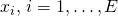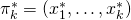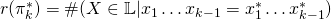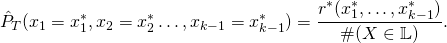# 3. A more sophisticated approach using Markov chains.

## 1. Generalize the Procedure

The very simple Educated Guess Procedure is not only very simple, the procedure is also very unrealistic. At most the assumption thatare independent and identically distributed random variables is not satisfied in practice. Consider for examplewhere observed, the probability thatshould then be higher then, even though our analyze reveals that assuming independence. Another recommendation in terms of “how to create a good password” given by experts is often to use the first letter of some Phrase. For example “I like to eat 10 red apples in the morning” will become “Ilte10raitm”. Look’s pretty random at first glance, however language is not random and thus such passwords are weaker (and easier to detect by the following method) than truly random passwords. To model these issues we introduce

(1)where the probability to observe a certain letter depends on the previousletters. One usually speaks in this context of a Markov chain of order, further it an be seen that our previous attempt is a special case of (1) where. In linguistics, such models are known as-grams.

## 2. Generate better “guessed” Passwords

To get estimates we will thus relay on-gram counting, to do so in advance we need some additional notation. Letbe a permutation with repetitions of lengthand withthe sum over all possible permutations is meant. An estimator for the probability of a specific permutationandcan then be derived by

(2)however, additional smoothing is needed especially if we assume an alphabet with many special characters because there is a chance that some-grams are not observed in our training sample. Such-grams will then be assigned with a probability 0 and will never be considered in any generation procedure, however in reality these-grams are for sure possible. We use Good-Turing smoothing, this means for a specific permutation, each countandthe number of k-grams observedtimes, an adjusted countis computed. A Good-Turing smoothed version of 2 is then given by

(3)Still we are facing possibly unrealistic assumptions, for example that the length of the password does not depend on the used letters. Another hard assumption is that we are always facing a-gram. A further generalization taking this issue into account would be to use a Variable-order Markov model with allows for different‘s depending on a given observation.

To start the Markov Chain we need an initial state, therefore randomly drawletters according with the following probabilities

(4)If we assume that,  then this will givepossible intal states according to a certain alphabet. We also need to construct a list of containing all possible transition probability given by (3) between the-grams. Combinatorics then tells us that there existpermutations. Using these lists we can then construct a Markov chain using the estimated probabilities. The Markov chain is then interrupted aftersteps whereist drawn, like in the previous approach, from.

This site uses Akismet to reduce spam. Learn how your comment data is processed.